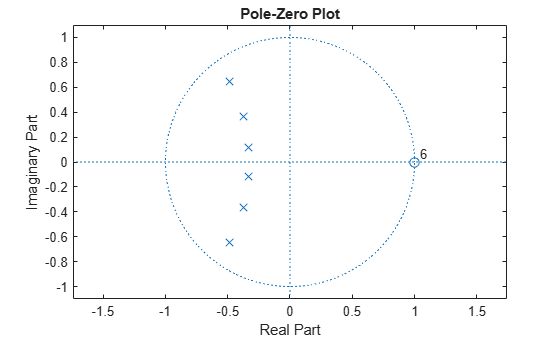Documentation

# isstable

Determine whether filter is stable

## Syntax

```flag = isstable(b,a) flag = isstable(sos) flag = isstable(d) flag = isstable(hs) flag = isstable(hs,'Arithmetic',arithtype) ```

## Description

`flag = isstable(b,a)` returns a logical output, `flag`, equal to `true` if the filter specified by numerator coefficients, `b`, and denominator coefficients, `a`, is a stable filter. If the poles lie on or outside the circle, `isstable` returns `false`. If the poles are inside the circle, `isstable` returns `true`.

`flag = isstable(sos)` returns `true` if the filter specified by second order sections matrix, `sos`, is stable. `sos` is a K-by-6 matrix, where the number of sections, K, must be greater than or equal to 2. Each row of `sos` corresponds to the coefficients of a second order (biquad) filter. The ith row of the `sos` matrix corresponds to ```[bi(1) bi(2) bi(3) ai(1) ai(2) ai(3)]```.

`flag = isstable(d)` returns `true` if the digital filter, `d`, is stable. Use `designfilt` to generate `d` based on frequency-response specifications.

```flag = isstable(hs)``` returns `true` if the filter System object™ `hs` is stable. You must have the DSP System Toolbox™ software to use this syntax.

```flag = isstable(hs,'Arithmetic',arithtype)``` analyzes the filter System object `hs` based on the specified `arithtype`. `arithtype` can be `'double'`, `'single'`, or `'fixed'`. When you specify `'double'` or `'single'`, the function performs double- or single-precision analysis. When you specify `'fixed'` , the arithmetic changes depending on the setting of the `CoefficientDataType` property and whether the System object is locked or unlocked. You must have the DSP System Toolbox software to use this syntax.

When you do not specify the arithmetic for non-CIC structures, the function uses double-precision arithmetic if the filter System object is in an unlocked state. If the System object is locked, the function performs analysis based on the locked input data type. CIC structures only support fixed-point arithmetic.

## Examples

collapse all

Design a sixth-order Butterworth highpass IIR filter using second order sections. Specify a normalized 3-dB frequency of 0.7. Determine if the filter is stable.

```[z,p,k] = butter(6,0.7,'high'); SOS = zp2sos(z,p,k); flag = isstable(SOS) ```
```flag = logical 1 ```
`zplane(z,p)`Redesign the filter using `designfilt` and check it for stability.

```d = designfilt('highpassiir','DesignMethod','butter','FilterOrder',6, ... 'HalfPowerFrequency',0.7); dflg = isstable(d)```
```dflg = logical 1 ```
`zplane(d)`Create a filter and determine its stability at double and single precision.

```b = [1 -0.5]; a = [1 -0.999999999]; act_flag1 = isstable(b,a)```
```act_flag1 = logical 1 ```
`act_flag2 = isstable(single(b),single(a))`
```act_flag2 = logical 0 ```